# Vary Parameter Values and Obtain Multiple Transfer Functions

This example shows how to use the `slLinearizer` interface to batch linearize a Simulink® model. You vary model parameter values and obtain multiple open-loop and closed-loop transfer functions from the model.

You can perform the same analysis using the `linearize` function. However, when you want to obtain multiple open-loop and closed-loop transfer functions, especially for models that are expensive to compile repeatedly, `slLinearizer` can be more efficient.

Since the parameter variations in this example do not affect the operating point of the model, you batch linearize the model at a single operating point. If your application uses parameter variations that affect the model operating point, first trim the model for each parameter value combination. For an example that uses the `linearize` function, see Batch Linearize Model at Multiple Operating Points Derived from Parameter Variations.

### Create `slLinearizer` Interface for Model

The `scdcascade` model used for this example contains a pair of cascaded feedback control loops. Each loop includes a PI controller. The plant models, `G1` (outer loop) and `G2` (inner loop), are LTI models.

Use the `slLinearizer` interface to analyze the inner-loop and outer-loop dynamics.

Open the model.

```mdl = 'scdcascade'; open_system(mdl) ```Use the `slLinearizer` function to create the interface.

```sllin = slLinearizer(mdl) ```
``` slLinearizer linearization interface for "scdcascade": No analysis points. Use the addPoint command to add new points. No permanent openings. Use the addOpening command to add new permanent openings. Properties with dot notation get/set access: Parameters : [] OperatingPoints : [] (model initial condition will be used.) BlockSubstitutions : [] Options : [1x1 linearize.LinearizeOptions] ```

The Command Window display shows information about the `slLinearizer` interface. In this interface, no parameters to vary are yet specified, so the `Paramaeters` property is empty.

### Vary Inner-Loop Controller Gains

For inner-loop analysis, vary the gains of the inner-loop PI controller block, C2. Vary the proportional gain (`Kp2`) and integral gain (`Ki2`) in the 15% range.

```Kp2_range = linspace(Kp2*0.85,Kp2*1.15,6); Ki2_range = linspace(Ki2*0.85,Ki2*1.15,4); [Kp2_grid, Ki2_grid] = ndgrid(Kp2_range,Ki2_range); params(1).Name = 'Kp2'; params(1).Value = Kp2_grid; params(2).Name = 'Ki2'; params(2).Value = Ki2_grid; sllin.Parameters = params; ```

`Kp2_range` and `Ki2_range` specify the sample values for `Kp2` and `Ki2`. To obtain a transfer function for each combination of `Kp2` and `Ki2`, use `ndgrid` and create a 6 x 4 parameter grid with grid arrays `Kp2_grid` and `Ki2_grid`. Configure the `Parameters` property of `sllin` with the structure `params`. This structure specifies the parameters to be varied and their grid arrays.

### Analyze Closed-Loop Transfer Function for the Inner Loop

The overall closed-loop transfer function for the inner loop is equal to the transfer function from `u1` to `y2`. To eliminate the effects of the outer loop, you can break the loop at `e1`, `y1m`, or `y1`. For this example, break the loop at `e1`.

Add `u1` and `y2` as analysis points, and `e1` as a permanent opening of `sllin`.

```addPoint(sllin,{'y2','u1'}); addOpening(sllin,'e1'); ```

Obtain the transfer function from `u1` to `y2`.

```r2yi = getIOTransfer(sllin,'u1','y2'); ```

`r2yi`, a 6 x 4 state-space model array, contains the transfer function for each specified parameter combination. The software uses the model initial conditions as the linearization operating point.

Because `e1` is a permanent opening of `sllin`, `r2yi` does not include the effects of the outer loop.

Plot the step response for `r2yi`.

```stepplot(r2yi); ```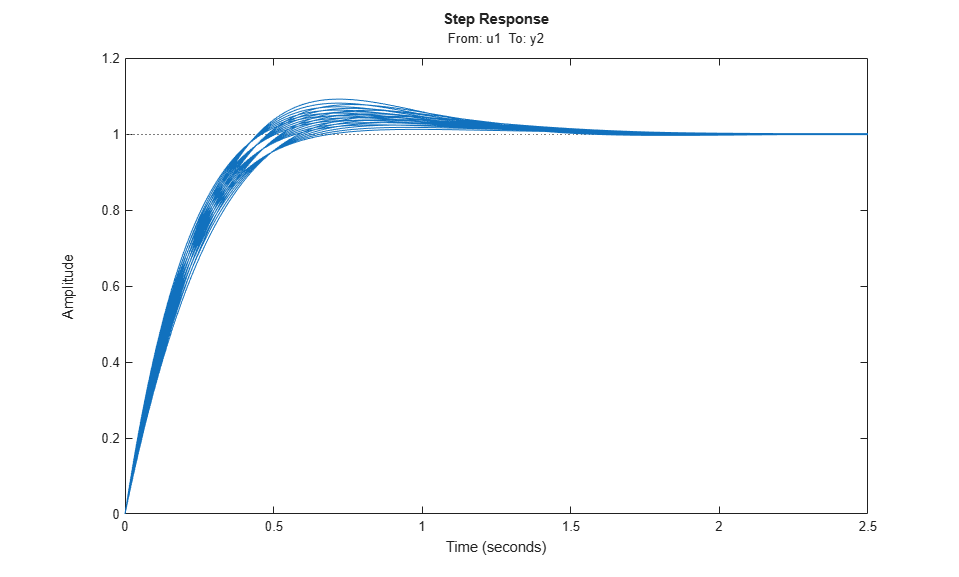The step response for all models varies in the 10% range and the settling time is less than 1.5 seconds.

### Analyze Inner-Loop Transfer Function at the Plant Output

Obtain the inner-loop transfer function at `y2`, with the outer loop open at `e1`.

```Li = getLoopTransfer(sllin,'y2',-1); ```

Because the software assumes positive feedback by default and `scdcascade` uses negative feedback, specify the feedback sign using the third input argument. Now,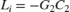. The `getLoopTransfer` command returns an array of state-space (`ss`) models, one for each entry in the parameter grid. The `SamplingGrid` property of `Li` matches the parameter values with the corresponding `ss` model.

Plot the bode response for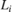.

```bodeplot(Li) ```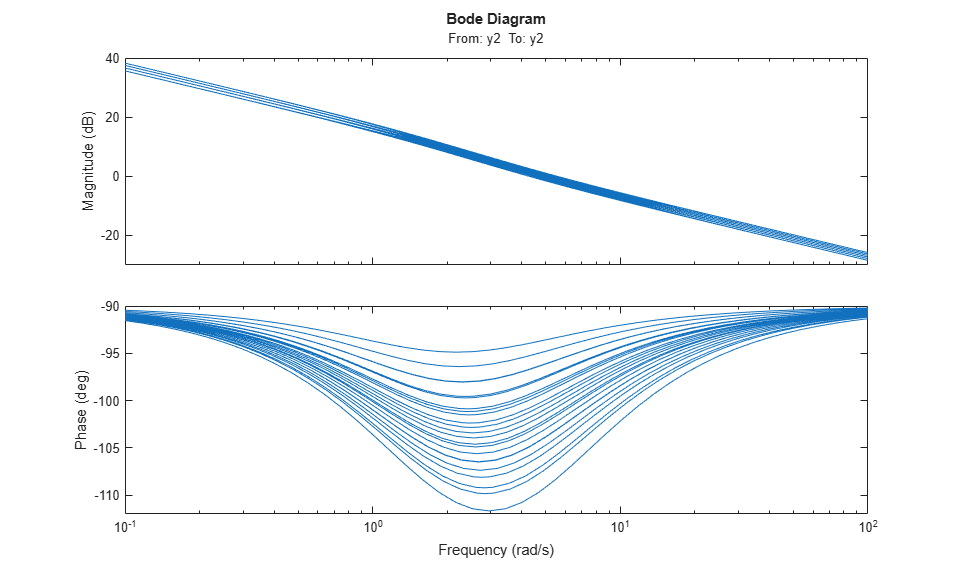The magnitude plot for all the models varies in the 3-dB range. The phase plot shows the most variation, approximately 20°, in the `[1 10]` rad/s interval.

### Vary Outer-Loop Controller Gains

For outer-loop analysis, vary the gains of the outer-loop PI controller block, C1. Vary the proportional gain (`Kp1`) and integral gain (`Ki1`) in the 20% range.

```Kp1_range = linspace(Kp1*0.8,Kp1*1.2,6); Ki1_range = linspace(Ki1*0.8,Ki1*1.2,4); [Kp1_grid, Ki1_grid] = ndgrid(Kp1_range,Ki1_range); params(1).Name = 'Kp1'; params(1).Value = Kp1_grid; params(2).Name = 'Ki1'; params(2).Value = Ki1_grid; sllin.Parameters = params; ```

Similar to the workflow for configuring the parameter grid for inner-loop analysis, create the structure, `params`, that specifies a 6 x 4 parameter grid. Reconfigure `sllin.Parameters` to use the new parameter grid. `sllin` now uses the default values for `Kp2` and `Ki2`.

### Analyze Closed-Loop Transfer Function from Reference to Plant Output

Remove `e1` from the list of permanent openings for `sllin` before proceeding with outer-loop analysis.

```removeOpening(sllin,'e1'); ```

To obtain the closed-loop transfer function from the reference signal, `r`, to the plant output, `y1m`, add `r` and `y1m` as analysis points to `sllin`.

```addPoint(sllin,{'r','y1m'}); ```

Obtain the transfer function from `r` to `y1m`.

```r2yo = getIOTransfer(sllin,'r','y1m'); ```

Plot the step response for `r2yo`.

```stepplot(r2yo) ```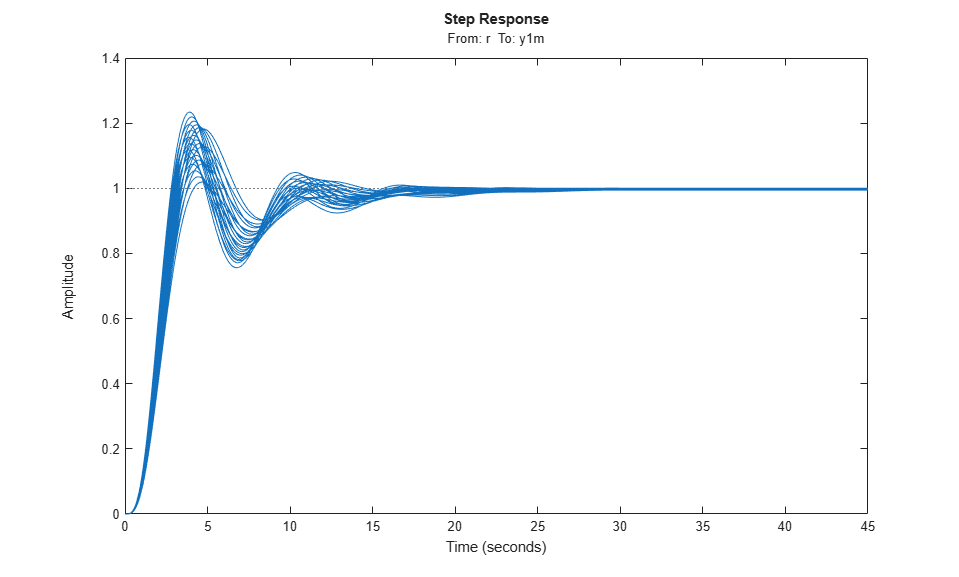The step response is underdamped for all the models.

### Analyze Outer-Loop Sensitivity at Plant Output

To obtain the outer-loop sensitivity at the plant output, add `y1` as an analysis point to `sllin`.

```addPoint(sllin,'y1'); ```

Obtain the outer-loop sensitivity at `y1`.

```So = getSensitivity(sllin,'y1'); ```

Plot the step response of `So`.

```stepplot(So) ```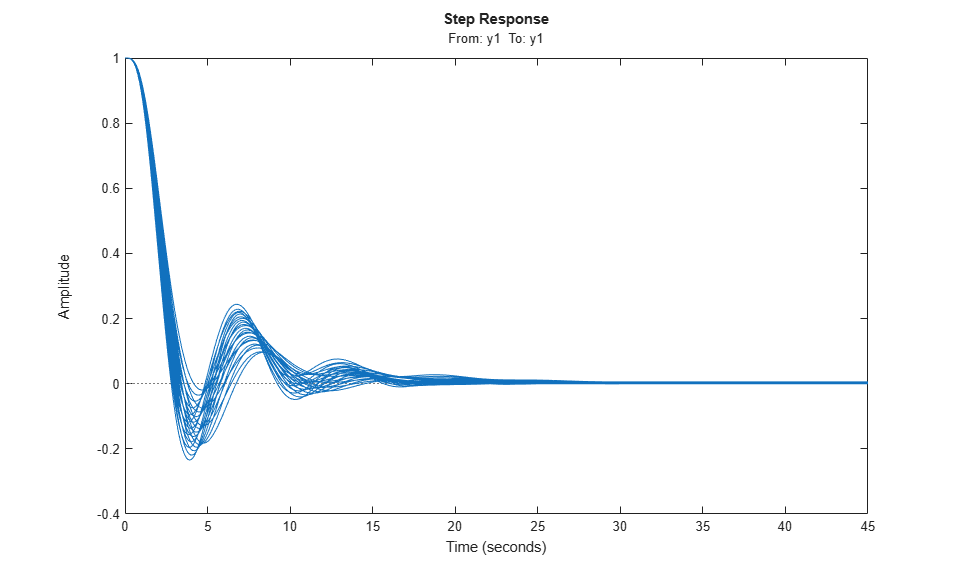The plot indicates that it takes approximately 15 seconds to reject a step disturbance at the plant output, `y1`.

### Obtain Linearization Offsets

When batch linearizing for parameter variations, you can obtain the linearization offsets that correspond to the linearization operating points. To do so, set the `StoreOffsets` linearization option in the `slLinearizer` interface.

```sllin.Options.StoreOffsets = true; ```

When you call a linearization function using `sllin`, you can return linearization offsets in the `info` structure.

```[r2yi,info] = getIOTransfer(sllin,'u1','y2'); ```

You can then use the offsets to configure an LPV System block. To do so, you must first convert the offsets to the required format. For an example that uses the `linearize` command, see LPV Approximation of Boost Converter Model.

```offsets = getOffsetsForLPV(info); ```

Close the model.

```bdclose(mdl) ```